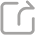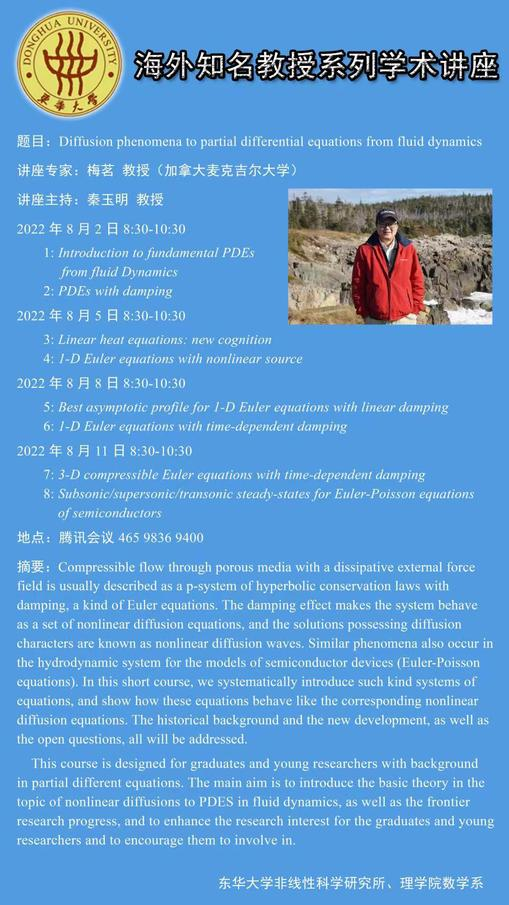分享至QQ空间 分享至朋友圈 分享至新浪微博

### PDE系列学术讲座：Diffusion phenomena to partial differential equations from fluid dynamics

Compressible flow through porous media with a dissipative external force field is usually described as a p-system of hyperbolic conservation laws with damping, a kind of Euler equations. The damping effect makes the system behave as a set of nonlinear diffusion equations, and the solutions possessing diffusion characters are known as nonlinear diffusion waves. Similar phenomena also occur in the hydrodynamic system for the models of semiconductor devices (Euler-Poisson equations). In this short course, we systematically introduce such kind systems of equations, and show how these equations behave like the corresponding nonlinear diffusion equations. The historical background and the new development, as well as the open questions, all will be addressed.

This course is designed for graduates and young researchers with background in partial different equations. The main aim is to introduce the basic theory in the topic of nonlinear diffusions to PDES in fluid dynamics, as well as the frontier research progress, and to enhance the research interest for the graduates and young researchers and to encourage them to involve in.